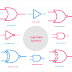In digital electronic circuits, logic gates are the basic fundamental component or building blocks. Every digital circuit, device consists of logic gates. Logic gates have one or multiple inputs and one output. Generally, logic gates are made of transistors. In this article, we are going to see the symbol of logic gates. After reading this article, you will know all types of logic gate symbols.

## AND Gate Symbol

An AND gate has a minimum of two inputs. The output of the AND gate will be high when both of the input is high. If any one input is low the output also be low. Here, you can see the symbol of AND Gate.

## OR Gate Symbol

An OR Gate also has a minimum of two inputs. The output of the OR Gate will be high when anyone input is high. The output will be low when both of the inputs is low. Here, you can see the symbol of OR Gate.

## NOT Gate Symbol

A NOT gate is also known as an inverter. It has a single input and a single output. When the input of the NOT gate is high the output will be low and vice versa. Here, you can see the symbol of NOT Gate.

## NAND Gate Symbol

NAND gate is the combination of AND Gate and NOT gate. It also has a minimum of two inputs and one output. The output of the NAND Gate will be high when anyone input is low. The output will be low when both of the inputs are high. Here, you can see the symbol of NAND Gate.

## NOR Gate Symbol

A NOR Gate is the combination of OR Gate and NOT Gate. NOR gate also have a minimum of two inputs and one output. The output of the NOR gate will be high when both of the input is low. The output of the NOR gate will be low when anyone input is low. Here, you can see the symbol of NOT Gate.

## XOR Gate Symbol

Here, you can see the symbol of XOR Gate. The XOR gate is also known as the Exclusive OR gate. It is a digital logic gate that performs an exclusive OR operation on two input signals. It has two inputs and one output. The output of the XOR gate is high (logic 1) only when the number of high inputs is odd. If both inputs are the same (either both high or both low), the output is low (logic 0).

## XNOR Gate Symbol

Here, you can see the symbol of XNOR Gate. The XNOR gate is also known as the Exclusive NOR gate. It is a digital logic gate that performs the opposite operation of the XOR gate. XNOR Gate also has two inputs and one output. It produces a high output (logic 1) when the number of high inputs is even. In other words, the output of the XNOR gate is high when both inputs are the same (either both high or both low), and low when the inputs differ.

## Buffer Symbol

A buffer is a type of logic gate that simply amplifies or repeats the input signal without any logical operation or inversion. The purpose of a buffer is to isolate or separate different parts of a circuit that may have different characteristics or voltage levels. A buffer is often represented by a symbol that resembles a triangle, with an input on one side and an output on the other, indicating the amplification or repetition of the input signal. You can see it looks like the NOT gate but the circle is not here.

Logic Gate Symbols - OR, AND, NOT, NOR, NAND, XOR, XNORReviewed by Author on November 02, 2021 Rating: 5